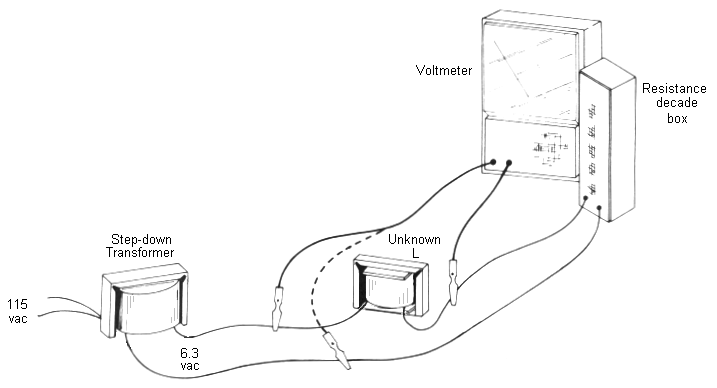# Measuring Inductance

If inductance is so important, how do you measure inductance?

Of course you can buy an inductance meter, but there are several problems. Inductance meters are not common; they are hard to find and expensive. Worse yet, they measure at very low current and unknown frequency. This can give misleading results when your coilgun has an iron projectile.

## Simple Method for Measuring Inductance

Absolute precision is not critical here, so you can use this setup to measure inductance.

You will need:

• 6.3v step-down transformer. Small transformers are good because they limit the maximum current.
• resistor decade box, such as found in college labs.Step 1. Get a resistor decade box, and set it at maximum resistance.

Step 2. Connect resistor decade box in series with the inductor, as shown above.

Step 3. With no power applied, connect one lead of the decade box to the outputs (6.3vac) of a step-down power transformer.

Step 4. Apply power to the primary (120vac at 60 Hz). Adjust the resistance until you measure the same voltage across both the resistor and inductor (or capacitor). You can do this by moving just one meter lead as shown by the dashed line.

Step 5. Read the resistance value from the decade box. This number is equal to the reactance (XL) of the device under test!

Step 6. Finally, calculate the inductance L using:

L = XL / (2 π f), and if your frequency f = 60 Hz, then:    L = XL / 377

 < Previous Page 4 of 6 Next >